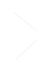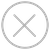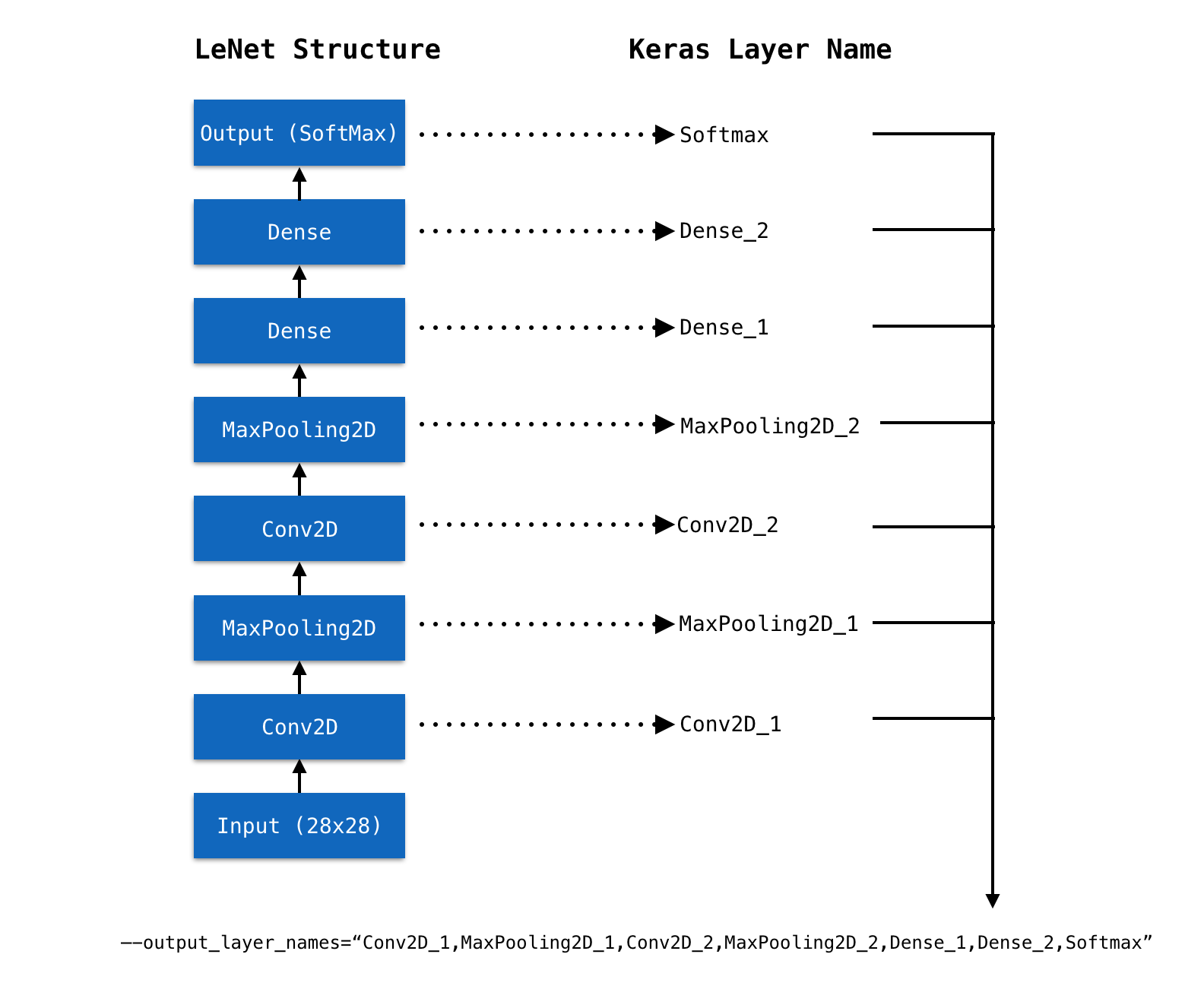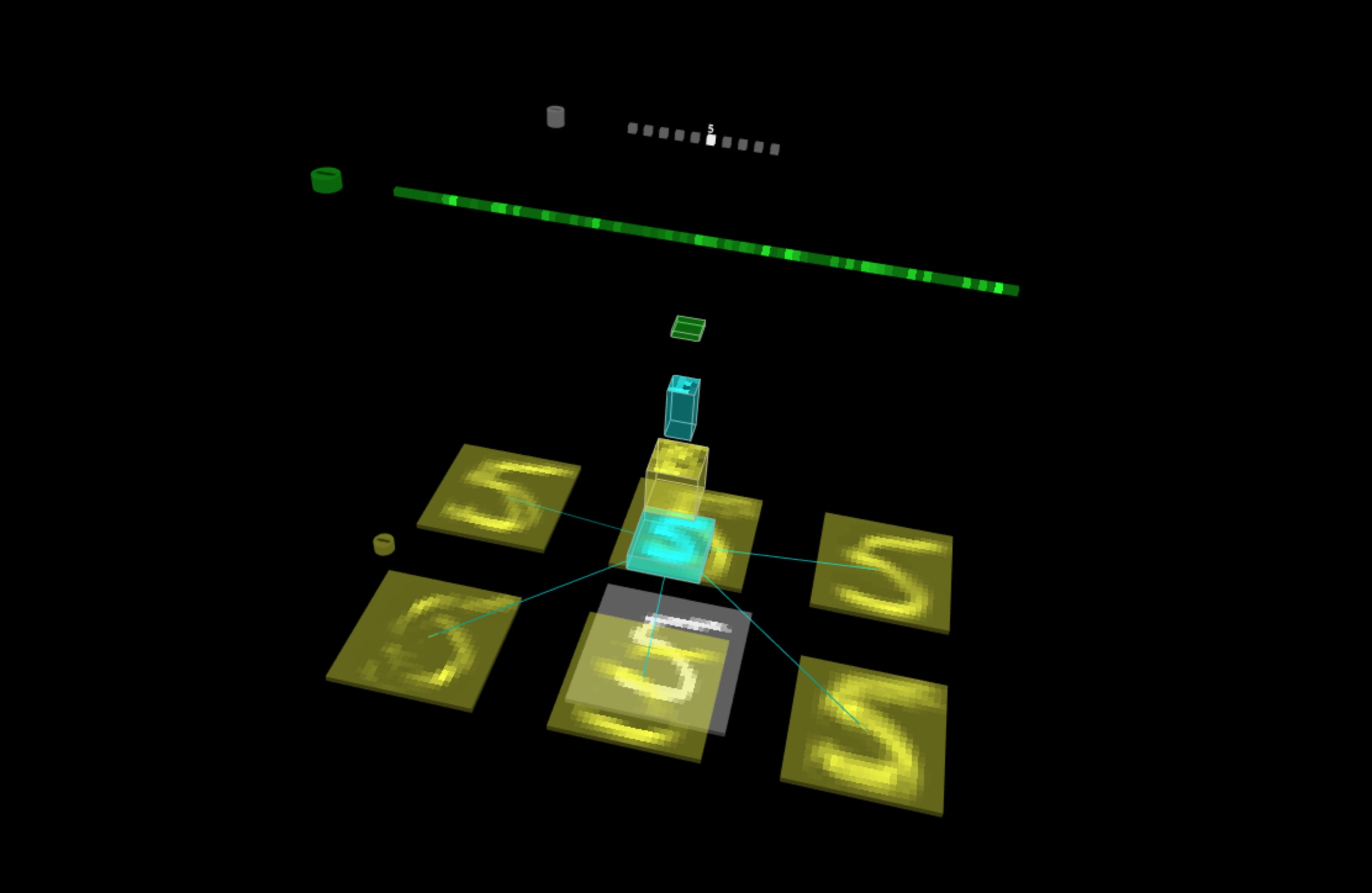TensorSpace.jsTensorSpace Converterfilter_center_focus Keras 模型
filter_center_focus TensorSpace 可视化代码

``````\$ tensorspacejs_converter \
--input_model_from="keras" \
--input_model_format="topology_weights_combined" \
--output_node_names="Conv2D_1,MaxPooling2D_1,Conv2D_2,MaxPooling2D_2,Dense_1,Dense_2,Softmax" \
./rawModel/combined/mnist.h5 \
./convertedModel/``````
wb_sunny注意:
• filter_center_focusinput_model_from 设置成 keras
• filter_center_focusinput_model_format 设置成 topology_weights_combined
• filter_center_focus.h5 文件的路径设置到 input_path 中。
• filter_center_focus 取出 Keras 模型的 Layer 名称 , 然后设置到 output_layer_names 中，如 图1 所示。
• filter_center_focus 以上 TensorSpace-Converter 预处理脚本将会在 convertedModel 文件夹中生成经过预处理的模型。在本教程中，我们已经生成了经过预处理的模型，模型文件可以在 这个文件夹 中找到。

``````\$ tensorspacejs_converter \
--input_model_from="keras" \
--input_model_format="topology_weights_separated" \
--output_node_names="Conv2D_1,MaxPooling2D_1,Conv2D_2,MaxPooling2D_2,Dense_1,Dense_2,Softmax" \
./rawModel/separated/topology.json,./rawModel/separated/weight.h5 \
./convertedModel/``````
wb_sunny注意:
• filter_center_focusinput_model_from 设置成 keras
• filter_center_focusinput_model_format 设置成 topology_weights_separated
• filter_center_focus 对于这种模型类型，因为有两个模型文件，在设置 TensorSpace-Converter 的 input_path 时，合并两个文件的路径，并用英文半角逗号“,”分开，将 .json 文件的路径在前，.h5 文件的路径在后。
• filter_center_focus 取出 Keras 模型的 Layer 名称 , 然后设置到 output_layer_names 中，如 图1 所示。
• filter_center_focus 以上 TensorSpace-Converter 预处理脚本将会在 convertedModel 文件夹中生成经过预处理的模型。在本教程中，我们已经生成了经过预处理的模型，模型文件可以在 这个文件夹 中找到。wb_sunny注意:
• filter_center_focus 我们将会得到2种不同类型的文件：
• flare 一份 model.json 文件：包含所得到的模型结构信息（包括中间层输出）。
• flare 一些 权重 文件：包含模型训练所得到的权重信息。权重文件的数量取决于模型的结构。

``````let model = new TSP.models.Sequential( modelContainer );

``````model.load( {